A.2 Wenzel-Kramers-Brillouin Method

The WKB method  is an approximative semiclassical approach to compute the stationary solution of the Schrödinger equation without struggling with the difficulties of a second order differential equation. Taking the time-independent, one-dimensional Schrödinger equation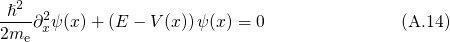as a starting point and inserting the ansatz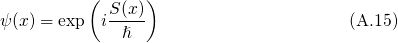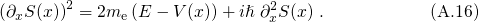Substituting the action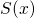by its expansion in power series of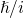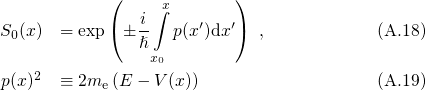for terms of the order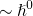and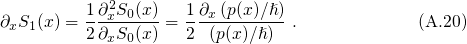for terms of the order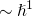. Integrating (A.20), one obtains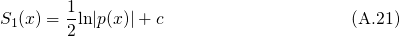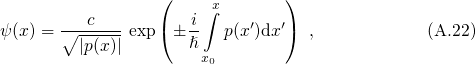where the integration spans from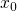to an arbitrary point.is also referred to as the classical turning point, where the particle energy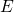equals the potential energy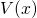. Note that close to this point, the WKB approximation breaks down and the expression for the wavefunction diverges since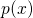in the denominator approaches zero. As a result, the wavefunction left and right to this point cannot be adjusted, which is the case at the discontinuity of the semiconductor-dielectric interface for instance. One way to overcome this problem is to apply Langer’s procedure , which is not presented here. The above formula also applies to classical forbidden regions where the particle energylies below the potential barrier.